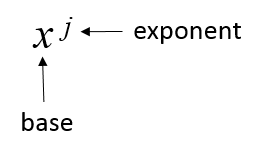# rules of exponents

## Quick guide

This is a summary of the rules of exponents material used in the xlf presentation series. It include numerical examples, and keystrokes for the Hewlett Packard 12C financial calculator.

### Exponents

A exponent is shown as a superscript to a base number - see figure 1.Fig 1. - In the expression $x^j$, $j$ is the exponent and $x$ is the base.

RPN keystrokes ►

Exponent ruleNumerical example
$x^0=1$
See Note 1
$5^0=1$
$\text{12c: }5\;[\text{Enter}]\;0\;[y^x]\;\text{returns} \;1$
$x^1=x$$4^1=4$
$\text{12c: }4\;[\text{Enter}]\;1\;[y^x]\;\text{returns} \;4$
$x^{-1}=1/x$$2^{-1}=1/2$
$\text{12c: }2\;[\text{Enter}]\;1\;[\text{CHS}]\;[y^x]\;\text{returns} \;0.5$
Product rule
$x^j x^k=x^{j+k}$
$x^2x^4=x^{2+4}=x^6$
$2^2\cdot2^4=4\cdot16=64; \quad 2^6=64$
$\text{12c: }2\;[\text{Enter}]\;2\;[y^x]\;2\;[\text{Enter}]\;4\;[y^x]\;[×]\;\text{returns}\;64$
Quotient rule
$x^j/x^k=x^{j-k}$
$x^7/x^3=x^{7-3}=x^4$
$5^7/5^3=5^{7-3}=5^4=625; \quad 78125/125=625$
$\text{12c: }5\;[\text{Enter}]\;7\;[y^x]\;5\;[\text{Enter}]\;3\;[y^x]\;[÷]\;\text{returns} \;625$
Power rule
$(x^j)^k=x^{jk}$
$(x^2)^4=x^{2\cdot4}=x^8$
$(4^2)^4=4^8=65536; \quad 16^4=65536$
$\text{12c: }4\;[\text{Enter}]\;2\;[y^x]\;\;4\;[y^x]\;\text{returns} \;65536$
$(xy)^j=x^jy^j$$(xy)^3=x^3y^3$
$(2\cdot3)^3=2^3\cdot3^3=8\cdot27=216; \quad 6^3=216$
$\text{12c: }2\;[\text{Enter}]\;3\;[×]\;3\;[y^x]\;\text{returns}\;216$
$(x/y)^j=x^j/y^j$$(x/y)^2=x^2/y^2$
$(4/5)^2=4^2/5^2=16/25=0.64; \quad(0.8)^2=0.64$
$\text{12c: }4\;[\text{Enter}]\;5\;[÷]\;2\;[y^x]\;\text{returns}\;0.64$
$x^{-j}=1/x^j$$x^{-3}=1/x^3$
$2^{-3}=1/2^3=1/8; \quad2^{-3}=0.125$
$\text{12c: }2\;[\text{Enter}]\;3\;[\text{CHS}]\;[y^x]\;\text{returns}\;0.125$

Note 1: $\dfrac{x^j} {x^j}=1$, thus by application of the quotient rule $x^{j-j}=x^0=1$

### The exponential function

The special case where the base $x$ is set to the mathematical constant $e, 2.71828$ is important in many areas of finance, such as interest rates in continuous time. The notation $e^x$ is called the exponential function, and is also written as $exp(x)$. From the exponent rules, $exp(1)=e=2.71828$, and $exp(0)=1$.

• Published: 23 July 2014
• Revised: Tuesday 25th of February 2020 - 04:44 PM, [Australian Eastern Standard Time (EST)]